# NCERT Exemplar Class 10 Science Chapter 1 Chemical Reactions and Equations Solutions

NCERT Exemplar Solutions for Class 10 Science Chapter 1 Chemical Reactions and equations covers all the important questions and answers as well as advanced level questions. It helps in learning all the fundamentals of chemical reactions, daily examples of chemical reactions, balancing of chemical reactions and writing of chemical reactions.

The NCERT Exemplar solutions for class 10 science is very important for board exams. NCERT Exemplar Solutions for Class 10 Science Chapter 1 Chemical Reactions and equations is provided by our experts. They prepared the best solutions which help the students in understanding the solutions in an easy way. This chapters also covers the other topics like type of chemical reactions, oxidation and reduction rusting and different preventive measures.

 Chapter Name Chapter 1 Chemical Reactions and Equations Book Title NCERT Exemplar for Class 10 Science Related Study NCERT Solutions for Class 10 Science Chapter 1 Chemical Reactions and EquationsRevision Notes for Class 10 Science Chapter 1 Chemical Reactions and EquationsMCQ for Class 10 Science Chapter 1 Chemical Reactions and EquationsImportant Questions for Class 10 Science Chapter 1 Chemical Reactions and Equations Topics Covered MCQShort Answers QuestionsLong Answers Questions

### Multiple Choice Questions

1. Which of the following is not a physical change?
(a) Boiling of water to give water vapour
(b) Melting of ice to give water
(c) Dissolution of salt in water
(d) Combustion of Liquefied Petroleum Gas (LPG)

Solution

(d) Combustion of Liquefied Petroleum Gas(LPG)

Combustion is always a chemical change because new compound is formed after burning and it is irreversible.

2. The following reaction is an example of a 4NH3 (g) + 5O2 (g) → 4NO(g) + 6H2O(g)
(i) displacement reaction
(ii) combination reaction
(iii) redox reaction
(iv) neutralisation reaction

(a) (i) and (iv)
(b) (ii) and (iii)
(c) (i) and (iii)
(d) (iii) and (iv)

Solution

(a) (i) and (iv)

The reaction provided is a mixture of displacement and redox reaction because in this reaction oxygen displace hydrogen in the ammonia. Here nitrogen is getting oxidized and oxygen is reduced.

3. Which of the following statements about the given reaction are correct?
3Fe(s) + 4H2O(g) → Fe3O4 (s) + 4H2 (g)

(i) Iron metal is getting oxidised
(ii) Water is getting reduced
(iii) Water is acting as reducing agent
(iv) Water is acting as oxidising agent

(a) (i), (ii) and (iii)
(b) (iii) and (iv)
(c) (i), (ii) and (iv)
(d) (ii) and (iv)

Solution

(d) (ii) and (iv)

Here, Oxygen combines with water to get oxidized. Oxygen is removed from water hence it is getting reduced. Water is providing oxygen and it acts as an oxidizing agent.

4. Which of the following are exothermic processes?
(i) Reaction of water with quick lime
(ii) Dilution of an acid
(iii) Evaporation of water
(iv) Sublimation of camphor (crystals)

(a) (i) and (ii)
(b) (ii) and (iii)
(c) (i) and (iv)
(d) (iii) and (iv)

Solution

(a) (i) and (ii)
Exothermic process are the one which release enormous amount of heat When water reacts with water and acid reacts with water it releases enormous amount of heat.

5. Three beakers labelled as A, B and C each containing 25 mL of water were taken. A small amount of NaOH, anhydrous CuSO4 and NaCl were added to the beakers A, B and C respectively. It was observed that there was an increase in the temperature of the solutions contained in beakers A and B, whereas in case of beaker C, the temperature of the solution falls. Which one of the following statement(s) is(are) correct?
(i) In beakers A and B, exothermic process has occurred.
(ii) In beakers A and B, endothermic process has occurred.
(iii) In beaker C exothermic process has occurred.
(iv) In beaker C endothermic process has occurred.

(a) (i) only
(b) (ii) only
(c) (i) and (iv)
(d) (ii) and (iii)

Solution

(c) (i) and (iv)
Exothermic processes will increase the temperature wherease exothermic processes will decrease the temperature.

6. A dilute ferrous sulphate solution was gradually added to the beaker containing acidified permanganate solution. The light purple colour of the solution fades and finally disappears. Which of the following is the correct explanation for the observation?

(a) KMnO4 is an oxidising agent, it oxidises FeSO4
(b) FeSO4 acts as an oxidising agent and oxidises KMnO4
(c) The colour disappears due to dilution; no reaction is involved
(d) KMnO4 is an unstable compound and decomposes in presence of FeSO4 to a colourless compound.

Solution

(a) KMnO4 is an oxidising agent, it oxidises FeSO4
In this reaction potassium permanganate is an oxidizing agent. Purple color appeared due to potassium permanganate when all the permanganate solution is utilized.

7. Which among the following is(are) double displacement reaction(s)?
(i) Pb + CuCl2 → PbCl2 + Cu
(ii) Na2SO4 + BaCl2 → BaSO4 + 2NaCl
(iii) C + O2 → CO2
(iv) CH4 + 2O2 → CO2 + 2H2O

(a) (i) and (iv)
(b) (ii) only
(c) (i) and (ii)
(d) (iii) and (iv)

Solution

(b) (ii) only
Here Sodium and Barium are displaced from each other's salts hence it is a double displacement reaction.

8. Which among the following statement(s) is (are) true? Exposure of silver chloride to sunlight for a long duration turns grey due to
(i) the formation of silver by decomposition of silver chloride
(ii) sublimation of silver chloride
(iii) decomposition of chlorine gas from silver chloride
(iv) oxidation of silver chloride

(a) (i) only
(b) (i) and (iii)
(c) (ii) and (iii)
(d) (iv) only

Solution

(a) (i) only

9. Solid calcium oxide reacts vigorously with water to form calcium hydroxide accompanied by liberation of heat. This process is called slaking of lime. Calcium hydroxide dissolves in water to form its solution called lime water. Which among the following is (are) true about slaking of lime and the solution formed?
(i) It is an endothermic reaction
(ii) It is an exothermic reaction
(iii) The pH of the resulting solution will be more than seven
(iv) The pH of the resulting solution will be less than seven

(a) (i) and (ii)
(b) (ii) and (iii)
(c) (i) and (iv)
(d) (iii) and (iv)

Solution

(b) (ii) and (iii)
When Solid calcium oxide reacts vigorously with water to form calcium hydroxide accompanied by liberation of heat. It proves the reaction is exothermic. pH of the solution will be more than 7 because oxides and hydorxides of metals are alkaline.

10. Barium chloride on reacting with ammonium sulphate forms barium sulphate and ammonium chloride. Which of the following correctly represents the type of the reaction involved?
(i) Displacement reaction
(ii) Precipitation reaction
(iii) Combination reaction
(iv) Double displacement reaction

(a) (i) only
(b) (ii) only
(c) (iv) only
(d) (ii) and (iv)

Solution

(c) (iv) only
Ammonium and barium are getting displaced from their respective salts. Hence this is a double displacement reaction.

11. Electrolysis of water is a decomposition reaction. The mole ratio of hydrogen and oxygen gases liberated during electrolysis of water is
(a) 1:1
(b) 2:1
(c) 4:1
(d) 1:2

Solution

(b) 2 : 1
1 Mole of water has 2 moles of hydrogen and 1 mole of water. Hence mole ration between hydrogen and oxygen is 2 : 1.

12. Which of the following is(are) an endothermic process(es)?
(i) Dilution of sulphuric acid
(ii) Sublimation of dry ice
(iii) Condensation of water vapours
(iv) Evaporation of water

(a) (i) and (iii)
(b) (ii) only
(c) (iii) only
(d) (ii) and (iv)

Solution

(d) (ii) and (iv)
Change of solid to gas or liquid to gas absorbs heat hence sublimation of dry ice and evaporation is an endothermic reaction.

13. In the double displacement reaction between aqueous potassium iodide and aqueous lead nitrate, a yellow precipitate of lead iodide is formed. While performing the activity if lead nitrate is not available, which of the following can be used in place of lead nitrate ?

(c) Ammonium nitrate
(d) Potassium sulphate

Solution

To get lead iodide we need a compound containing lead hence Ammonium nitrate and Potassium sulphate are ruled out. Lead sulphate is insoluble hence it cannot be used so the answer is (b) Lead acetate.

14. Which of the following gases can be used for storage of fresh sample of an oil for a long time ?
(a) Carbon dioxide or oxygen
(b) Nitrogen or oxygen
(c) Carbon dioxide or helium
(d) Helium or nitrogen

Solution

(d) Helium or nitrogen
Oxygen cannot be used as it is an oxidizing agent. Helium can be used as it is an inert gas. Nitrogen is less reactive and it is cheaper than Helium. In most cases nitrogen is used in packet to prevent rancidity.

15. The following reaction is used for the preparation of oxygen gas in the laboratory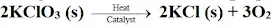Which of the following statement (s) is (are) correct about the reaction?
(a) It is a decomposition reaction and endothermic in nature.
(b) It is a combination reaction.
(c) It is a decomposition reaction and accompanied by release of heat .
(d) It is a photochemical decomposition reaction and exothermic in nature.
Solution

(a) It is a decomposition reaction and endothermic in nature.

Potassium chlorate decomposes to give potassium chloride and oxygen. This is a decomposition reaction which is endothermic in nature.

16. Which one of the following processes involve chemical reactions?

(a) Storing of oxygen gas under pressure in a gas cylinder

(b) Liquefaction of air

(c) Keeping petrol in a china dish in the open

(d) Heating copper wire in presence of air at high temperature

Solution

(d) Heating copper wire in presence of air at high temperature

There is no involvement of chemical reaction in the first three options given here. Copper undergoes oxidation reaction to form copper oxide when it is heated in presence of air at high temperature.

17. In which of the following chemical equations, the abbreviations represent the correct states of the reactants and products involved at reaction temperature ?

(a) 2H2 (l) + O2 (l) → 2H2O(g)
(b) 2H2 (g) + O2 (l) → 2H2O(l)
(c) 2H2 (g) + O2 (g) → 2H2O(l)
(d) 2H2 (g) + O2 (g) → 2H2O(g)

Solution

(c) 2H2 (g) + O2 (g) → 2H2O(l)

Hydrogen and oxygen are gases at room temperature whereas water is liquid. Hence option (c) represents correct states of reactants and products.

18. Which of the following are combination reactions ?

2. MgO + H2O → Mg(OH)2

3. 4Al + 3O2 → 2Al2O3
4. Zn + FeSO4 → ZnSO4 + Fe

(a) 1 and 3
(b) 3 and 4
(c) 2 and 4
(d) 2 and 3

Solution

(d) 2 and 3

Here, two reactants react to form a single product hence option ii) and iii) represents combination reactions.

19. Write the balanced chemical equations for the following reactions and identify the type of reaction in each case.
(a) Nitrogen gas is treated with hydrogen gas in the presence of a catalyst at 773K to form ammonia gas.
(b) Sodium hydroxide solution is treated with acetic acid to form sodium acetate and water.
(c) Ethanol is warmed with ethanoic acid to form ethyl acetate in the presence of concentrated H2SO4  .
(d) Ethene is burnt in the presence of oxygen to form carbon dioxide, water and releases heat and light.

Solution

Combination reaction

(b) NaOH(aq) + CH3COOH(aq) ⟶ CH3COONa(aq) + H2O(l)
Neutralisation reaction

(d) C2H4(g) + 3O2(g) → 2CO2(g) + 2H2O(g) + Heat + Light
Oxidation reaction

20. Write the balanced chemical equations for the following reactions and identify the type of reaction in each case.
(a) Thermit reaction, iron (III) oxide reacts with aluminium and gives molten iron and aluminium oxide. (b) Magnesium ribbon is burnt in an atmosphere of nitrogen gas to form solid magnesium nitride.
(c) Chlorine gas is passed in an aqueous potassium iodide solution to form potassium chloride solution and solid iodine.
(d) Ethanol is burnt in air to form carbon dioxide, water and releases heat.

Solution

(a) Fe2O3(s) + 2Al(s) → Al2O3(s) + 2Fe(l) + Heat
Single displacement reaction

(b) 3Mg(s) + N2(g) → Mg3N2(s)
Combination reaction

(c) 2KI(aq) +Cl2(g) →2KCl(aq) + I2(s)
Single displacement reaction

(d) C2H5OH(l) + 3O2(g) → 2CO2(g) + 3H2O(l) + Heat
Combustion reaction

21. Complete the missing components/variables given as x and y in the following reactions
(a) Pb(NO3)2 [aq] + 2Kl[aq] → Pbl2[X] + 2KNO[y]
(b) Cu[s] + 2Ag NO3 [aq] → Cu[NO3]2 [aq] + x[s]
(c) Zn[s] + H2SO4[aq] → ZnSO4[x] + H2[y]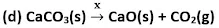Solution

(a) Pb(NO3)2 [aq] + 2Kl[aq] → Pbl2[s] + 2KNO[aq]
(b) Cu[s] + 2Ag NO3 [aq] → Cu[NO3]2 [aq] + 2Ag[s]
(c) Zn[s] + H2SO4[aq] → ZnSO4[aq] + H2[g]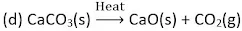22. Which among the following changes are exothermic or endothermic in nature?
(a) Decomposition of ferrous sulphate
(b) Dilution of sulphuric acid
(c) Dissolution of sodium hydroxide in water
(d) Dissolution of ammonium chloride in water

Solution

(a) Decomposition of ferrous sulphate - Endothermic reaction

(b) Dilution of sulphuric acid -Exothermic reactions

(c) Dissolution of sodium hydroxide in water -Exothermic reactions

(d) Dissolution of ammonium chloride in water-Endothermic reaction

23. Identify the reducing agent in the following reactions

(a) 4NH3 + 5O2 → 4NO + 6H2O
(b) H2O + F2 → HF + HOF
(c) Fe2O3 + 3CO → 2Fe + 3CO2
(d) 2H2 + O2 → 2H2O

Solution

(a) NH3 Ammonia
(b) F2 – Fluorine
(c) CO – Carbon momnoxide
(d) H2 – Hydrogen

24. Identify the oxidising agent (oxidant) in the following reactions

(a) Pb3O4 + 8HCl → 3PbCl2 + Cl2 + 4H2O
(b) 2Mg + O2 → 2MgO
(c) CuSO4 + Zn → Cu + ZnSO4
(d) V2O5 + 5Ca → 2V + 5CaO
(e)3Fe + 4H2O → Fe3O4 +4H2
(f) CuO + H2 → Cu + H2O

Solution

(a) Pb3O4
(b) O2
(c) CuSO4
(d) V2O5
(e) H2O
(f) CuO

25. Write the balanced chemical equations for the following reactions
(a) Sodium carbonate on reaction with hydrochloric acid in equal molar concentrations gives sodium chloride and sodium hydrogencarbonate.
(b) Sodium hydrogencarbonate on reaction with hydrochloric acid gives sodium chloride, water and liberates carbon dioxide.
(c) Copper sulphate on treatment with potassium iodide precipitates cuprous iodide (Cu2 I2 ), liberates iodine gas and also forms potassium sulphate.

Solution

(a) Na2CO3 + HCl → NaCl + NaHCO3
(b) NaHCO3 + HCl → NaCl + H2O + CO2
(c) 2CuSO4 + 4Kl → 2K2SO4 + CU2l2 + I2

26. A solution of potassium chloride when mixed with silver nitrate solution, an insoluble white substance is formed. Write the chemical reaction involved and also mention the type of the chemical reaction ?

Solution

KCl(aq) + AgNO3(aq) → AgCl(s) + KNO3(aq)
It is a double displacement and precipitation reaction.

27. Ferrrous sulphate decomposes with the evolution of a gas having a characteristic odour of burning sulphur. Write the chemical reaction involved and identify the type of reaction.

Solution

2FeSO4 → Fe2O3 + SO2 + SO3
This is a decomposition reaction.

28. Why do fire flies glow at night ?

Solution

A chemical reaction is produced in inside the bodies of fireflies. This chemical reaction allows them to glow at night. Oxygen combines with calcium, ATP and luciferin in the presence of an enzyme called luciferase. This results in bioluminescence.

29. Grapes hanging on the plant do not ferment but after being plucked from the plant can be fermented. Under what conditions do these grapes ferment ? Is it a chemical or a physical change ?

Solution

Grapes are living when attached to the plants and therefore their own immune system prevents fermentation. In the plucked grapes the microbes can grow and under anaerobic conditions these can be fermented. This is a chemical change.

30. Which among the following are physical or chemical changes ?
(a) Evaporation of petrol
(b) Burning of Liquefied Petroleum Gas(LPG)
(c) Heating of an iron rod to red hot.
(d) Curdling of milk
(e) Sublimation of solid ammonium chloride

Solution

(a) Evaporation of petrol -Physical change

(b) Burning of Liquefied Petroleum Gas (LPG) -Chemical change

(c) Heating of an iron rod to red hot -Physical change

(d) Curdling of milk -Chemical change

(e) Sublimation of solid ammonium chloride-Physical change

31. During the reaction of some metals with dilute hydrochloric acid, following observations were made.
(a) Silver metal does not show any change
(b) The temperature of  the reaction mixture rises when aluminium (Al) is added.
(c) The reaction of sodium metal is found to be highly explosive
(d) Some bubbles of a gas are seen when lead(Pb) is reacted with the acid.
Explain these observations giving suitable reasons.

Solution

(a) As silver lies in low reactive series of metals, there will be no reaction between silver and dilute HCl.

(b) It is an exothermic reaction, therefore the temperature increases.

(c) Sodium is highly reactive metals. An exothermic reaction takes place between sodium and atmospheric oxygen which results in temperature increase.

(d) Hydrogen gas is produced when lead reacts with acid. This is responsible for the formation of bubbles.

32. A substance X, which is an oxide of a group 2 element, is used intensively in the cement industry. This element is present in bones also. On treatment with water it forms a solution which turns red litmus blue. Identify X and also write the chemical reactions involved.

Solution

Compound X is Calcium oxide.
CaO + H2O → Ca(OH)2

33. Write a balanced chemical equation for each of the following reactions and also classify them.
(a) Lead acetate solution is treated with dilute hydrochloric acid to form lead chloride and acetic acid solution.
(b) A piece of sodium metal is added to absolute ethanol to form sodium ethoxide and hydrogen gas.
(c) Iron (III) oxide on heating with carbon monoxide gas reacts to form solid iron and liberates carbons dioxide gas.
(d) Hydrogen sulphide gas reacts with oxygen gas to form solid sulphur and liquid water.

Solution

(a) Pb(CH3COO)2 + 2HCI - PbCl2 + CH3COOH
This is a Double Displacement reaction.
(b) 2Na + 2C2H5OH + 2C2H5ONa+ H2
This is a Displacement reaction.
(c) Fe2O3 + 3CO + 2Fe + 3CO2
This is a redox reaction.
(d) 2H2S + O→ 2s + 2H2O
This is a replacement reaction.

34. Why do we store silver chloride in dark coloured bottles?

Solution

On exposure to sunlight, silver chloride may decompose according to the following reaction.
2AgCl → 2Ag + Cl2
Thus, it is stored in dark coloured bottles.

35. Balance the following chemical equations and identify the type of chemical reaction.

(a) Mg(s) + Cl2(g) → MgCl2(s)

(b) HgO(s) → Hg(l) + O2(g)

(c) Na(s) + S(s) → Na2S(s)

(d) TiCl4(l) + Mg(s) → Ti(s) + MgCl2(s)

(e) CaO(s) + SiO2(s) → CaSiO3(s)

(f) H2O2(l) →H2O(l) + O2(g)

Solution

(a) Mg(s) + Cl2(g) → MgCl2(s)
Combination reaction or Synthesis reaction.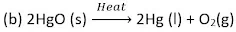Decomposition reaction

(d) TiCl4(l) + Mg(s) → Ti(s) + 2MgCl2 (s)
Displacement reaction

(e) CaO(s) + SIO2(s) + CaSIO3(s)
Synthesis reaction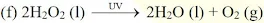Decomposition reaction

36. A magnesium ribbon is burnt in oxygen to give a white compound X accompanied by emission of light. If the burning ribbon is now placed in an atmosphere of nitrogen, it continues to burn and forms a compound Y.
(a) Write the chemical formulae of X and Y.
(b) Write a balanced chemical equation, when X is dissolved in water.

Solution

2Mg + O₂ → 2MgO
(a) Compound X → MgO [ Magnesium Oxide ]
Compound Y → Mg₃N₂ [ Magnesium Nitride ]

(b) When magnesium oxide is dissolved in water, magnesium hydroxide is formed.
MgO + H₂O → Mg(OH)₂

37. Zinc liberates hydrogen gas when reacted with dilute hydrochloric acid, whereas copper does not. Explain why?

Solution

In the activity series of metals, zinc is above hydrogen whereas copper is below hydrogen. This is the reason why zinc displaces hydrogen from dilute hydrochloric acid, while copper does not.

Zn + HCl → ZnCl2+ H2

Cu + HCl → No reaction

38. A silver article generally turns black when kept in the open for a few days. The article when rubbed with toothpaste again starts shining.
(a) Why do silver articles turn black when kept in the open for a few days? Name the phenomenon involved.
(b) Name the black substance formed and give its chemical formula.

Solution

(a) Silver reacts with H2S present in the atmosphere to form a black colour compound silver sulphide. This phenomenon is called corrosion.

(b) The black substance formed is silver sulphide.
2Ag+ H2S → Ag2S + H2

39. On heating blue coloured powder of copper (II) nitrate in a boiling tube, copper oxide (black), oxygen gas and a brown gas X is formed
(a) Write a balanced chemical equation of the reaction.
(b) Identity the brown gas X evolved.
(c) Identity the type of reaction.
(d) What could be the pH range of aqueous solution of the gas X?

Solution

(b) The brown gas X evolved is nitrogen dioxide (NO2).

(c) This is a thermal decomposition reaction.

(d) As nitrogen dioxide is and oxide of a non-metal, it dissolves in water to form acidic solution. Therefore, pH of this solution is less than 7.

40. Give the characteristic tests for the following gases
(a) CO₂
(b) SO₂
(c) O₂
(d) H₂
Solution

The characteristic test are as follows:

(a) Lime water turns milky when Carbon dioxide (CO2) gas is passed through it due to the formation of insoluble calcium carbonate.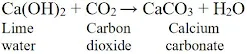(b) Sulphur dioxide (SO2) gas when passed through acidic potassium permanganate solution (purple in colour) turns it colourless because SO2 is a strong reducing agent.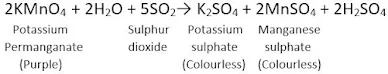When sulphur dioxide gas is passed through acidic dichromate solution (orange in colour), the solution turns green because sulphur dioxide is a strong reducing agent.

(c) Burning of match stick near oxygen makes it burn even more brightly.

(d) Hydrogen (H2) gas burns with a pop sound when a burning candle is brought near it.

41. What happens when a piece of
(a) zinc metal is added to copper sulphate solution?
(b) aluminium metal is added to dilute hydrochloric acid?
(c) silver metal is added to copper sulphate solution?
Also, write the balanced chemical equation if the reaction occurs.

Solution

(a) Zinc is more reactive than copper. Therefore zinc displaces copper from its solution and zinc sulphate solution is obtained.
Zn(s) + CuSo4(aq) → ZnSo4(aq)+ Cu(s)
This is an example of displacement reaction.

(b) Aluminium being more reactive displaces hydrogen from dilute hydrochloric acid solution. Hydrogen gas is evolved in the reaction.
2Al(s)+ 6HCl(aq) → 2AlCl3(aq)+ 3H2

(c) Silver metal is less reactive than copper. Therefore, silver cannot displace copper from its salt solution. Hence, no reaction occurs.
Ag (s) + CuSO4(aq) → No reaction

42. What happens when zinc granules are treated with dilute solution of H2SO4 , HCl, HNO3 , NaCl and NaOH, also write the chemical equations if reaction occurs.

Solution

The reaction of zinc granules with
(a) Dilute H2SO4
Displacement reaction takes place when zinc granules react with dil H2SO4 leading to the formation of ZnSO4 and H2 gas
Zn(s) + H2SO4 (aq) → ZnSO4(aq) + H2(g)

(b) Dilute HCl Displacement reaction takes place when zinc granules react with dil HCl leading to the formation of ZnCl2 and H2 gas
Zn(s) + 2HCl(aq) → ZnCl2(aq) + H2(g)

(c) Dilute HNO3
Reaction with dilute HNO3 is different as compared to other acids because nitric acid is an oxidising agent and it oxidises H2 gas evolved to H2O.
4 Zn(s) + 10HNO3(aq) → 4 Zn(NO3)2(aq) + 5 H2O(l) + N2O(g)

(d) NaCl solution
Zn(s) + NaCl (aq) → No reaction

(e) NaOH solution
Zn(s) + 2 NaOH (aq) → Na2ZnO2(aq) + H2

43. On adding a drop of barium chloride solution to an aqueous solution of sodium sulphite, white precipitate is obtained.
(a) Write a balanced chemical equation of the reaction involved
(b) What other name can be given to this precipitation reaction?
(c) On adding dilute hydrochloric acid to the reaction mixture, white precipitate disappears. Why?

Solution

(a) BaCl2 + Na2SO3 → BaSO3 + 2NaCl

(b) This precipitation reaction is also known as double displacement reaction.

(c) When dilute HCl tis added to this reaction mixture, Barium chloride, Sulphur dioxide and water are formed. Barium chloride is a soluble substance which will make the white precipitate disappear.
BaSO3 + HCl → BaCl3 + SO2 + H2O.

44. You are provided with two containers made up of copper and aluminium. You are also provided with solutions of dilute HCl, dilute HNO3 , ZnCl2 and H2O. In which of the above containers these solutions can be kept ?

Solution

(A) When solutions are kept in copper container:
(a) Dilute HCl
HCl can be kept in copper container as copper does not react with dilute HCl.
(b) Dilute HNO3
Nitric acid is a strong oxidising agent and reacts with copper vessel, therefore HNO3 cannot be kept in copper container.
(c) ZnCl2
No displacement reaction occurs as zinc is more reactive than copper (Cu). Therefore, ZnCl2 can be keptin copper container.
(d) H2O Copper does not react with water. Therefore, H2O can be kept in copper container.

(B) When solutions are kept in aluminium containers
(a) Dilute HCl
Aluminium reacts with dilute HCl to form its salt and hydrogen gas. Therefore, dilute HCl cannot be kept in aluminium containers.
2Al + 6HCl → 2AlCl3 + 3H2
(b) Dilute HNO3
Dilute HNO3oxidises aluminium to form a layer of Al2O3 and therefore dilute HNO3 cannot be kept in aluminium containers.
(c) ZnCl2
Aluminium is more reactive than zinc and therefore it displaces zinc ion from the solution. Therefore, ZnCl2solution cannot be kept in aluminium containers.
2Al + 3 ZnCl2 → 2 AlCl3 + 3Zn
(d) H2O Aluminium does not react with cold or hot water. Therefore, water can be kept in aluminium containers. Aluminium is attacked by steam to form aluminium oxide and hydrogen
2Al (s) + 3H2O (g) → Al2O3(s) + 3H2(g)